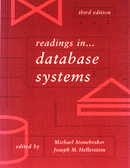Readings in Database Systems, 3rd EditionStonebraker & Hellerstein, eds.

# Query Processing

### A quick primer on the relational algebra

• selection
• projection
• cartesian product
• join (in particular, theta-join)

### Unary Operations

• sorting: see Knuth, or Shapiro paper.  We will study high-performance parallel sorting later.  Uses?
• hashing: a minor variant of hybrid-hash join works well. Uses?

### Binary Operations (i.e. Joins)

Join algorithms apply to almost any form of combining multiple collections.

Some commonly used join variants (alternative logical algebra operators):

• semi-join: R semi-join S ~= R join remove-dups(S) projected to the columns of R
• S basically serves as a filter
• logically, selection is a "virtual semijoin"
• outer join: R outer-join S: compute R join S, and for each tuple of R that has no match send it to the output with the S columns filled in with NULLs. Left, Right, and Full Outer Joins.
• intersection & difference

These logical algebra operators can be implemented as minor variations on the same join algorithms!

The "Guy Lohman Test" for join operators:

• does the operator work for joining 3 inputs without storing the output of 2 of the inputs?

### Nested Loops Join

```for each tuple r of R
for each tuple s of S
if rs satisfies join predicate
output rs  ```

R is the outer relation (left)

S is the inner relation (right)

• works for any join predicate
• inner input must be stored

### Refinement 1: Block Nested Loops Join

```for each block BR of R
for each tuple s of S
for each tuple r of BR such that rs satisfies join predicate
output rs  ```

Further refinements to nested loops:

• load R in chunks of M-K pages
• "pin" K pages of the inner into memory
• alternate scan direction on inner ("boustrophedonism")

### Refinement 2: Index Nested Loops Join

```for each tuple r of R
probe index over S;
output all s s.t. rs satisfies join predicate;  ```

Notes:

• Still called "nested loops", S is still referred to as the "inner" relation
• any join predicate can be supported if the index supports the predicate!
```        SELECT cities.name
FROM cities, forests
WHERE cities.boundary overlaps forests.boundary;  ```
• can convert all nested-loops joins to index-nested-loops by indexing inner on the fly

### (Sort-)Merge Join

Works for equijoin, "band" joins

we will assume here you know how to do a 2-pass sort [see Knuth or Shapiro]

idea: if R & S are sorted on the join column(s), we can "simply" merge them

But duplicates complicate things (as usual).

 R S R join S 1 1 1,1 2 2 2,2 2 4 2,2 3 5 5,5 5 5 5,5
```sort R;
sort S;
R.open();
S.open();
r = R.next();
s = S.next();
while (r != NULL && s != NULL) {
while (r.c < s.c)
r = R.next();
if (r.c = s.c) {
while (r.c = s.c) {
output rs;
r = R.next();
}
"rewind" r to first tuple of R where r.c = s.c;
s = S.next();
}
while (r.c > s.c)
s = S.next();
}  ```

Refinement: do merging of R & S while merging runs from each.

• Requires enough buffers for merging both R and S simultaneously.

Note: Sort-merge is symmetric, so "outer", "inner", "left", "right" are arbitrary

### Classic Hash Join

Works for equijoin only

Let R be the smaller relation

```Hash R into VM;
for each tuple of S
probe hashtable over R and output all rs s.t. s.c = r.c  ```
• In the spirit of indexed nested-loop join, S is "outer" (left), and R is "inner" (right)
• Warning: at least one important paper calls S the right relation, R the left!!!
• I prefer to call S left/outer, R right/inner
• note that hash join is very similar to nested-loop + index-on-the-fly (which Sybase used to do.)  If we call the probing relation there the "outer", we should do the same here!
• Safest to refer to "building" and "probing" relations

### Simple Hash Join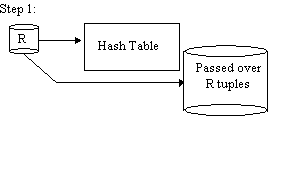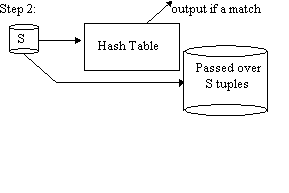Repeat steps 1 and 2 with R, S replaced by the passed over tuples.

• Great when R is small
• Works for memory of any size

• you tell me!

### Grace Hash Join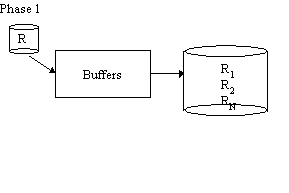Phase 1 is repeated with S in place of R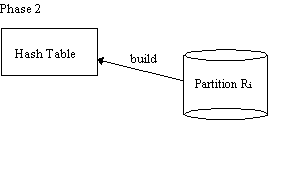THEN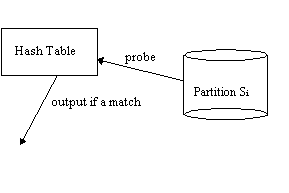• works well for big R, esp. when RAM is just big enough for output buffers of R

• you tell me!

### Hybrid Hash

Original paper: DeWitt, Katz, Olken, Shapiro, Stonebraker, Wood, SIGMOD '84.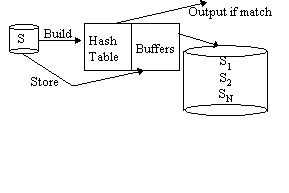Phase 2 as in Grace Join

• As good as simple hash for small R
• As good as Grace for big R
• If RAM is bigger than # of output buffers, improves on Grace

• you tell me

Handling Partition Overflow:

• If a partition of R overflows, recursively partition it, along with the corresponding bucket of S
• Note that size of S does not affect level of recursion!
• Makes hash-join particularly effective if |R| << |S| (compare to sort-merge)

Variation: Virtual Memory

• This is a Shapiro detail that is mostly ignored today...
• Define Hot Set as those pages which should be "pinned" in memory
• In our case, the hot set is the current hashtable in memory
• Any other memory (e.g. partitions) could be managed by VM!
• Problems:
• LRU paging leaves the "wrong end" of partitions in memory
• LRU screws up phase 2© 1998, Joseph M. Hellerstein.  Last modified 08/18/98. Feedback welcomed.